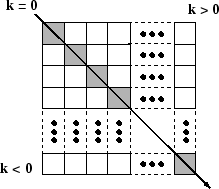Documentation

# diag

Create diagonal matrix or get diagonal elements of matrix

## Syntax

``D = diag(v)``
``D = diag(v,k)``
``x = diag(A)``
``x = diag(A,k)``

## Description

example

````D = diag(v)` returns a square diagonal matrix with the elements of vector `v` on the main diagonal.```

example

````D = diag(v,k)` places the elements of vector `v` on the `k`th diagonal. `k=0` represents the main diagonal, `k>0` is above the main diagonal, and `k<0` is below the main diagonal.```

example

````x = diag(A)` returns a column vector of the main diagonal elements of `A`.```

example

````x = diag(A,k)` returns a column vector of the elements on the `k`th diagonal of `A`.```

## Examples

collapse all

Create a 1-by-5 vector.

`v = [2 1 -1 -2 -5];`

Use `diag` to create a matrix with the elements of `v` on the main diagonal.

`D = diag(v)`
```D = 5×5 2 0 0 0 0 0 1 0 0 0 0 0 -1 0 0 0 0 0 -2 0 0 0 0 0 -5 ```

Create a matrix with the elements of `v` on the first super diagonal (`k=1`).

`D1 = diag(v,1)`
```D1 = 6×6 0 2 0 0 0 0 0 0 1 0 0 0 0 0 0 -1 0 0 0 0 0 0 -2 0 0 0 0 0 0 -5 0 0 0 0 0 0 ```

The result is a 6-by-6 matrix. When you specify a vector of length `n` as an input, `diag` returns a square matrix of size `n+abs(k)`.

Get the elements on the main diagonal of a random 6-by-6 matrix.

`A = randi(10,6)`
```A = 6×6 9 3 10 8 7 8 10 6 5 10 8 1 2 10 9 7 8 3 10 10 2 1 4 1 7 2 5 9 7 1 1 10 10 10 2 9 ```
`x = diag(A)`
```x = 6×1 9 6 9 1 7 9 ```

Get the elements on the first subdiagonal (`k=-1`) of `A`. The result has one fewer element than the main diagonal.

`x1 = diag(A,-1)`
```x1 = 5×1 10 10 2 9 2 ```

Calling `diag` twice returns a diagonal matrix composed of the diagonal elements of the original matrix.

`A1 = diag(diag(A))`
```A1 = 6×6 9 0 0 0 0 0 0 6 0 0 0 0 0 0 9 0 0 0 0 0 0 1 0 0 0 0 0 0 7 0 0 0 0 0 0 9 ```

## Input Arguments

collapse all

Diagonal elements, specified as a vector. If `v` is a vector with `N` elements, then `diag(v,k)` is a square matrix of order `N+abs(k)`.

`diag([])` returns an empty matrix, `[]`.

Data Types: `single` | `double` | `int8` | `int16` | `int32` | `int64` | `uint8` | `uint16` | `uint32` | `uint64` | `logical` | `char`
Complex Number Support: Yes

Input matrix. `diag` returns an error if ```ndims(A) > 2```.

`diag([])` returns an empty matrix, `[]`.

Data Types: `single` | `double` | `int8` | `int16` | `int32` | `int64` | `uint8` | `uint16` | `uint32` | `uint64` | `logical` | `char`
Complex Number Support: Yes

Diagonal number, specified as an integer. `k=0` represents the main diagonal, `k>0` is above the main diagonal, and `k<0` is below the main diagonal.

For an m-by-n matrix, `k` is in the range $\left(-m+1\right)\le k\le \left(n-1\right)\text{\hspace{0.17em}}\text{\hspace{0.17em}}.$